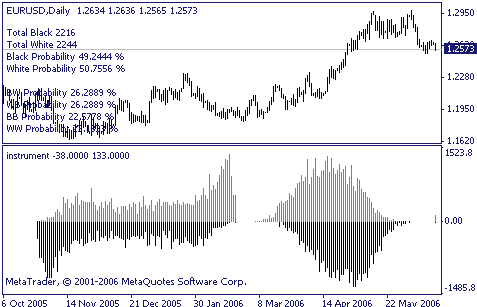### Introduction

Trading is, first of all, a calculus of probabilities. The proverb about idleness being an engine for progress reveals us the reason why all those indicators and trading systems have been developed. It comes that the major of newcomers in trading study "ready-made" trading theories. But, as luck would have it, there are some more undiscovered market secrets, and tools used in analyzing of price movements exist, basically, as those unrealized technical indicators or math and stat packages. Thanks awfully to Bill Williams for his contribution to the market movements theory. Though, perhaps, it's too early to rest on oars.

### Keeping Statistics

We can ask ourselves: "What color of candlesticks prevails in the one-hour chart for EURUSD?" We can start to count black ones noting every new hundred thereof in the block, then count white ones. But we can also write about a dozen of code lines, which will do this automatically. Basically, everything is logical and there is nothing unusual here. However, let us find an answer to the above question. First of all, let us simplify the candlestick color identification:

```bool isBlack(int shift)
{
if(Open[shift] > Close[shift])
return (true);
return (false);
}
//+------------------------------------------------------------------+
bool isWhite(int shift)
{
if(Open[shift] < Close[shift])
return (true);
return (false);
}
//+------------------------------------------------------------------+```

Using the code already written, we will continue the experiment.

```//EXAMPLE 1
//Calculate black and white candles
double BlackCandlesCount = 0;
double WhiteCandlesCount = 0;
double BProbability = 0;

for(int i = 0; i < Bars - 1; i++)
{
if(isBlack(i) == true)
BlackCandlesCount++;

if(isWhite(i) == true)
WhiteCandlesCount++;
}

BProbability = BlackCandlesCount / Bars;```

The result is interesting and quite predictable: 52.5426% of 16000 candles are white. Using the MQL4 compiler, we can also solve a problem of candles cyclicity. For example, if a black candle has been closed, what is the probability of forming a white one? This, of course, depends on a great variety of factors, but let us refer to statistics.

```//EXAMPLE 2
//Calculate seqences of 1st order
//BW means after black going white candle
double BW = 0;
double WB = 0;
double BB = 0;
double WW = 0;

for(i = Bars; i > 0; i--)
{
if(isBlack(i) && isWhite(i-1))
BW++;
if(isWhite(i) && isBlack(i-1))
WB++;
if(isBlack(i) && isBlack(i-1))
BB++;
if(isWhite(i) && isWhite(i-1))
WW++;
}```

The result obtained:
- White followed by Black - 23.64 %
- Black followed by White - 23.67 %
- White followed by White - 21.14 %
- Black followed by Black - 20.85 %

As we can see, the probability that a candle will be followed by a candle of the same color is a bit less than that of the opposite color.

Using MQL4 and having historical data, a trader can make some more profound market researches. The terminal allows drawing histograms. We will use this function to draw the candle color distribution according to values of indicators WPR and RSI.

```//EXAMPLE 3.1
//Build histogram by RSI
//RSI min/max - 0/100

double RSIHistogramBlack;
double RSIHistogramWhite;

for(i = Bars; i > 0; i--)
{
int rsi_val = iRSI(NULL,0,12,PRICE_CLOSE,i);
if(isWhite(i))
RSIHistogramWhite[rsi_val]++;
if(isBlack(i))
RSIHistogramBlack[rsi_val]++;
}
for(i = 0; i < 100; i++)
{
ExtMapBuffer1[i] = RSIHistogramBlack[i];
ExtMapBuffer2[i] = -RSIHistogramWhite[i];
}

//EXAMPLE 3.2
//Build histogram by %R
//%R min/max - 0/-100

double WPRHistogramBlack;
double WPRHistogramWhite;

for(i = Bars; i > 0; i--)
{
int wpr_val = iWPR(NULL,0,12,i);
int idx = MathAbs(wpr_val);
if (isWhite(i))
WPRHistogramWhite[idx]++;
if (isBlack(i))
WPRHistogramBlack[idx]++;
}```Anyway, it would be more objective, instead of counting black and white candlesticks, to keep statistics of profitable and losing trades with different values of StopLoss and TakeProfit. The procedure below will be helpful for this purpose:

```int TestOrder(int shift, int barscount, int spread, int tp, int sl, int operation)
{
double open_price = Close[shift];

open_price  = open_price + (Point * spread);

if (operation == OP_SELL)
open_price  = open_price - (Point * spread);

for (int i = 0; i<barscount; i++)
{
{
//sl
if (Low[shift-i] <= open_price - (Point * sl) )
return (MODE_STOPLOSS);
//tp
if (High[shift-i] >= open_price + (Point * tp) )
return (MODE_TAKEPROFIT);
}

if (operation == OP_SELL)
{
//sl
if (High[shift-i] >= open_price + (Point * sl) )
return (MODE_STOPLOSS);
//tp
if (Low[shift-i] <= open_price - (Point * tp) )
return (MODE_TAKEPROFIT);
}

}
return (MODE_EXPIRATION);
}```

I am sure that the results will be surprising for you. Kohonen's maps, Gaussian distribution, Hurst coefficient will astonish you even more. Basically, there are many astonishing things. The main thing is not to forget about the essence and sense of trading.

### Conclusion

Basically, every trader uses his or her own trading techniques. Of course, nothing will prevent him or her to represent the effectiveness of his or her system pictorially, analyze it and utilize in trading. No result is a result, too. Knowledge got by the trader will just enhance his or her trading productivity.